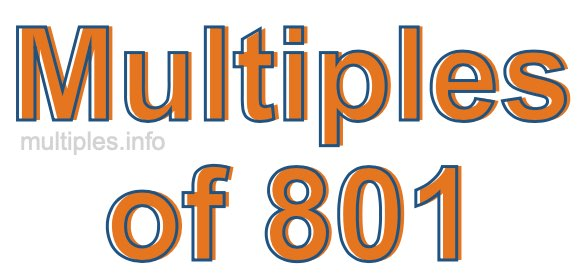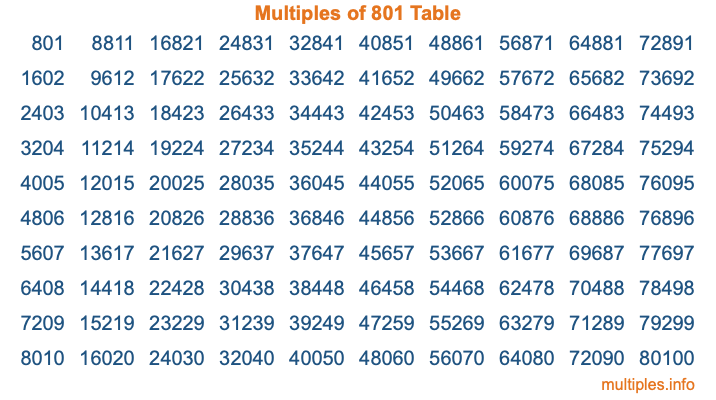Multiples of 801Welcome to the Multiples of 801 page. Here we will first teach you everything you will ever need to know about the multiples of 801, and then give you a study guide summary of everything we taught you to make sure you remember it all. Use this page to look up facts and learn information about the multiples of 801. This page will make you a multiples of eight hundred one expert!

Definition of Multiples of 801
Multiples of 801 are all the numbers that when divided by 801 equal an integer. Each of the multiples of 801 are called a multiple. A multiple of 801 is created by multiplying 801 by an integer.

Therefore, to create a list of multiples of 801, you start with 1 multiplied by 801, then 2 multiplied by 801, then 3 multiplied by 801, and so on for as long as you want. Thus, the list of the first five multiples of 801 is 801, 1602, 2403, 3204, and 4005. To see a larger list of multiples of 801, see the printable image of Multiples of 801 further down on this page. We also have a category where you can choose any nth multiple of 801.

Multiples of 801 Checker
The Multiples of 801 Checker below checks to see if any number of your choice is a multiple of 801. In other words, it checks to see if there is any number (integer) that when multiplied by 801 will equal your number. To do that, we divide your number by 801. If the the quotient is an integer, then your number is a multiple of 801.

Is  a multiple of 801?

Least Common Multiple of 801 and ...
A Least Common Multiple (LCM) is the lowest multiple that two or more numbers have in common. This is also called the smallest common multiple or lowest common multiple and is useful to know when you are adding our subtracting fractions. Enter one or more numbers below (801 is already entered) to find the LCM.

Check out our LCM Calculator if you need more details about the Least Common Multiple or if you need the LCM for different numbers for adding and subtraction fractions.

nth Multiple of 801
As we stated above, 801 is the first multiple of 801, 1602 is the second multiple of 801, 2403 is the third multiple of 801, and so on. Enter a number below to find the nth multiple of 801.

th multiple of 801

Multiples of 801 vs Factors of 801
801 is a multiple of 801 and a factor of 801, but that is where the similarities end. All postive multiples of 801 are 801 or greater than 801. All positive factors of 801 are 801 or less than 801.

Below is the beginning list of multiples of 801 and the factors of 801 so you can compare:

Multiples of 801: 801, 1602, 2403, 3204, 4005, etc.

Factors of 801: 1, 3, 9, 89, 267, 801

As you can see, the multiples of 801 are all the numbers that you can divide by 801 to get a whole number. The factors of 801, on the other hand, are all the whole numbers that you can multiply by another whole number to get 801.

It's also interesting to note that if a number (x) is a factor of 801, then 801 will also be a multiple of that number (x).

Multiples of 801 vs Divisors of 801
The divisors of 801 are all the integers that 801 can be divided by evenly. Below is a list of the divisors of 801.

Divisors of 801: 1, 3, 9, 89, 267, 801

The interesting thing to note here is that if you take any multiple of 801 and divide it by a divisor of 801, you will see that the quotient is an integer.

Multiples of 801 Table
Below is an image of the first 100 multiples of 801 in a table. The table is in chronological order, column by column. The first column has the first ten multiples of 801, the second column has the next ten multiples of 801, and so on.The Multiples of 801 Table is also referred to as the 801 Times Table or Times Table of 801. You are welcome to print out our table for your studies.

Negative Multiples of 801
Although not often discussed or needed in math, it is worth mentioning that you can make a list of negative multiples of 801 by multiplying 801 by -1, then by -2, then by -3, and so on, to get the following list of negative multiples of 801:

-801, -1602, -2403, -3204, -4005, etc.

Multiples of 801 Summary
Below is a summary of important Multiples of 801 facts that we have discussed on this page. To retain the knowledge on this page, we recommend that you read through the summary and explain to yourself or a study partner why they hold true.

There are an infinite number of multiples of 801.

A multiple of 801 divided by 801 will equal a whole number.

801 divided by a factor of 801 equals a divisor of 801.

The nth multiple of 801 is n times 801.

The largest factor of 801 is equal to the first positive multiple of 801.

801 is a multiple of every factor of 801.

801 is a multiple of 801.

A multiple of 801 divided by a divisor of 801 equals an integer.

801 divided by a divisor of 801 equals a factor of 801.

Any integer times 801 will equal a multiple of 801.

Multiples of a Number
Here you can get the multiples of another number, all with the same attention to detail as we did for multiples of 801 on this page.

Multiples of
Multiples of 802
Did you find our page about multiples of eight hundred one educational? Do you want more knowledge? Check out the multiples of the next number on our list!